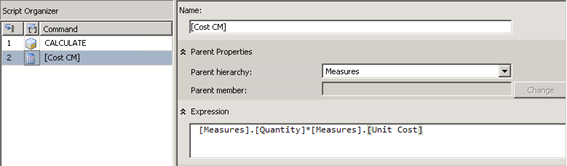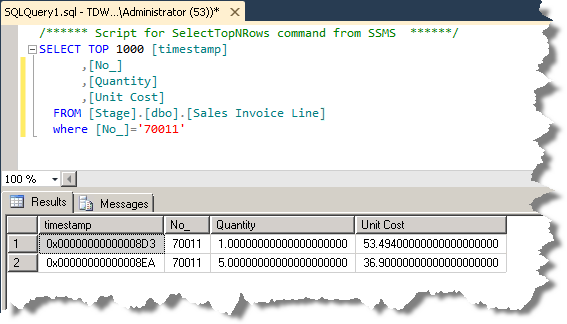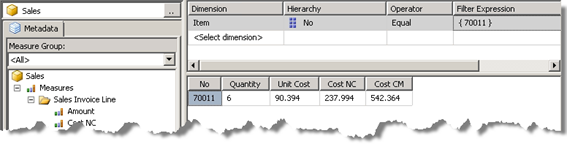# Best Practices: Named Calculations vs. Calculated Members

At first, when deciding whether to use Named Calculation or Calculated Members it may seem that both can be used in all situations and that will produce the same results. However, there is an important difference.

The difference lies hidden in this statement from the beginning of this chapter:

• “…Named calculations are calculated during processing whereas calculated members are calculated at query time…”

In other words: The results from Calculated Members will always be based on data that has already been processed and potentially summarized.

Let us demonstrate this with an example:

Suppose we want to calculate the Cost in two ways:

• Cost NC = Quantity x Unit Cost, as a Named Calculation.
• Cost CM = Quantity x Unit Cost, as a Calculated Member.

We have already done the Cost as a Named Calculation, and we only need to

create the Cost as a Calculated Member:Before processing the cube with this new Calculated Member, let us have a look at the Sales Invoice Line table in the SQL Server Management Studio. The following query shows that the Item with product number 70011 has two entries in the Sales Invoice Line table. Notice the different Quantities and Unit Costs.Now, go back to the cube, process it and have a look at the data in the cube browser. Add a filter to show data for Item 70011 only. This will produce one summarized result for Item 70011. Notice that both Quantity and Unit Cost has been summarized.

Also, notice that the two calculated results for Cost NC and Cost CM are different.The reason for the different results lies in the time these results have been calculated: Before or after processing of data.

In effect the two calculations have been made in this way:

• Cost NC = 1 x 53.494 + 5 x 36.9 = 237.994, which is correct!
• Cost CM = (1 + 5) x (53.494 + 36.9) = 542.364, which is wrong!

However, in other situations, when you actually want to base your calculations on summarized data, Calculated Members will produce the correct answer, while Named Calculations will be wrong.